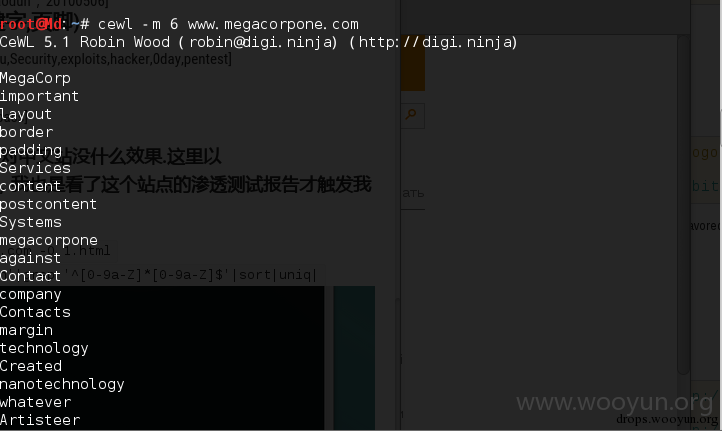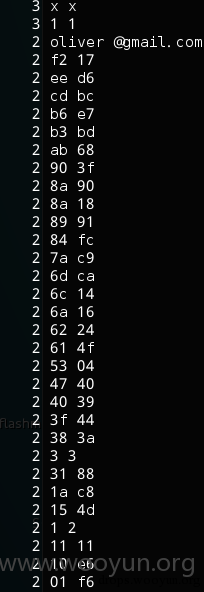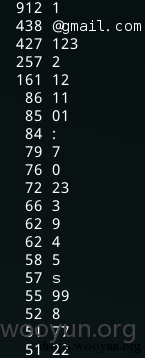# 0x00 研究范围

• 在WEB渗透中可能使用某个关键字为密码核心的密码(Mail,Vpn,后台登陆等)

# 0x01 实际数据分析

• Gmail 500W明文密码
• 个人以往渗透实例
• 美国姓名top2000

# 0x02 密码结构

• 前缀
• 关键字
• 连接符
• 后缀### 1.)关键字

• URL (二级域名,跟域名)
• keyword=[drop,wooyun,wooyun.org]
• 域名注册信息 (邮件,姓名,时间)
• keyword=[xssshell,fangxiaodun，20100506]
• 网站内容 （标题,关键字,页脚)
• keyword=[WooYun,zhishiku,Security,exploits,hacker,0day,pentest]
• 常用密码关键字

``````[email protected]/* <![CDATA[ */!function(t,e,r,n,c,a,p){try{t=document.currentScript||function(){for(t=document.getElementsByTagName('script'),e=t.length;e--;)if(t[e].getAttribute('data-cfhash'))return t[e]}();if(t&#038;&#038;(c=t.previousSibling)){p=t.parentNode;if(a=c.getAttribute('data-cfemail')){for(e='',r='0x'+a.substr(0,2)|0,n=2;a.length-n;n+=2)e+='%'+('0'+('0x'+a.substr(n,2)^r).toString(16)).slice(-2);p.replaceChild(document.createTextNode(decodeURIComponent(e)),c)}p.removeChild(t)}}catch(u){}}()/* ]]&gt; */:wget www.megacorpone.com -O 1.html
[email protected]/* <![CDATA[ */!function(t,e,r,n,c,a,p){try{t=document.currentScript||function(){for(t=document.getElementsByTagName('script'),e=t.length;e--;)if(t[e].getAttribute('data-cfhash'))return t[e]}();if(t&#038;&#038;(c=t.previousSibling)){p=t.parentNode;if(a=c.getAttribute('data-cfemail')){for(e='',r='0x'+a.substr(0,2)|0,n=2;a.length-n;n+=2)e+='%'+('0'+('0x'+a.substr(n,2)^r).toString(16)).slice(-2);p.replaceChild(document.createTextNode(decodeURIComponent(e)),c)}p.removeChild(t)}}catch(u){}}()/* ]]&gt; */:cat 1.html|tr ' ' '\n'|grep '^[0-9a-Z]*[0-9a-Z]\$'|sort|uniq|
``````### 2.)前缀与后缀

• 500W密码中有11561条密码是用用户名做关键字且排除了用户名为密码和用户名重复为密码。
• 11561条密码中有791条是前缀加关键字的模式• 11561条密码中有2308条是前缀加关键字加链接符加后缀的模式• 11561条密码中有8462条是关键字加后缀的模式• 连续性 字符连续的递增
• 重复性 字符重复
• 规则性 利用字符体现某种规则
• 键盘规则
• 寓意规则
• 应付性 满足系统强制要求

``````1234567890
qwertyuiopasdfghjklzxcvbnm
``````
• 键盘的设计是根据人体工程学设计的，最方便的键就是使用频率最高的键。玩过魔兽世界中pve无脑冰法的应该知道，【寒冰箭】基本都放数字1键，因为在抛弃字母键后，无名指按1键最方便，且连续按下123也最快捷。所以在 应付性 下满足系统要求（密码不为纯数字或纯字符）以字母做为关键字的密码，单数字前缀概率最大的为1
• 寓意规则 1(最大）,6(顺的寓意）,8（发的寓意）
• 键盘规则 ①中档键>右手>食指 J
• 寓意规则 以名字首字母为寓意 A``````~!@#\$%^&*()-_=+[{]}\|;:'",<.>/?
``````
• 键盘规则 在输出字符的时候需要按住Shift键
• 左手小指按Shift键 _
• 左手大拇指按Shift键 ！
• 寓意规则 @ 像a,邮件代表符号，易记忆

• 连续性 连续性字符大于2位，小于等于6位
• 123,abc,!@#
• 重复性 重复性字符串大于1位小于等于3位
• 888,qq,..
• 规则性
• 键盘规则
• qwe,147
• 寓意规则
• woshi,520,@))*，名词top100,短语top100
• @））* == shift（2008）

### 3.）链接符

• @ 寓意规则
• _ 应付性
• & 键盘规则### 4.)组合方式

• 前缀+关键字
• 前缀多应付性和寓意性
• 前缀+关键字+链接符+后缀
• 关键字多为寓意性
• 关键字+后缀
• ALL
• 关键字+链接符+后缀
• 后缀多为键盘规则和连续性